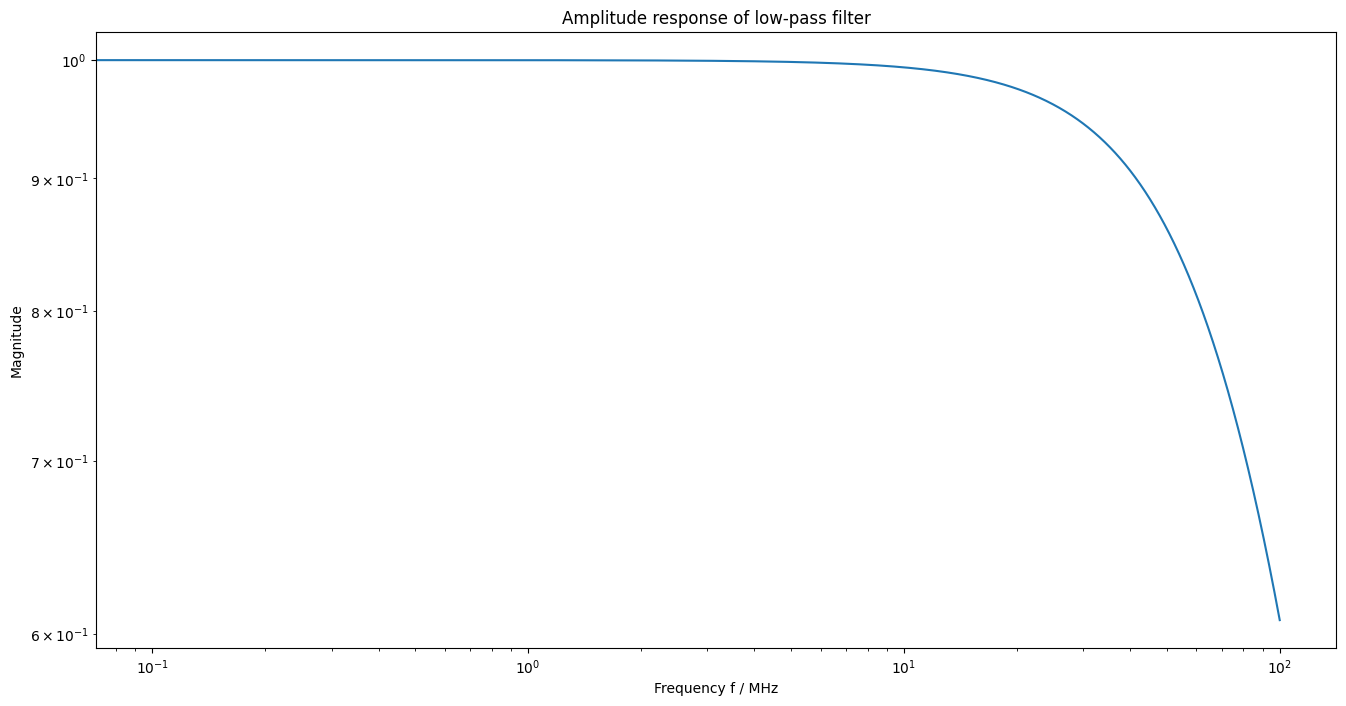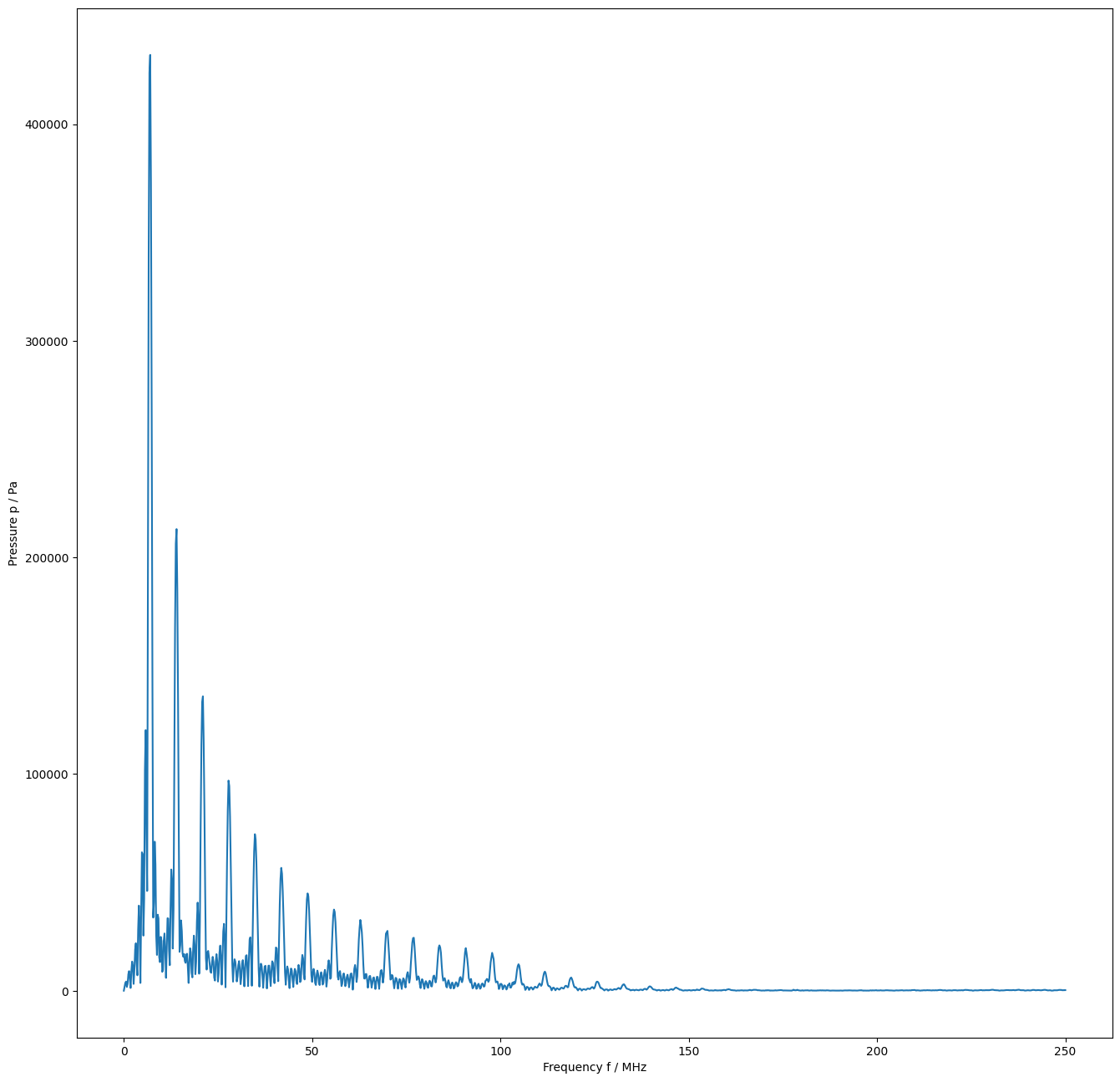# 6. Regularized deconvolution¶

:

%pylab inline

Populating the interactive namespace from numpy and matplotlib

:

from meas_data_preprocessing import *
from hydrophone_data_preprocessing import *
from PyDynamic.uncertainty.propagate_DFT import DFT_deconv, GUM_iDFT, DFT_multiply
from helper_methods import *
from regularization_bias import *

/home/ludwig10/code/envs/PyDynamic_tutorials-py38/lib/python3.8/site-packages/PyDynamic/identification/fit_filter.py:24: DeprecationWarning: The module *identification* will be combined with the module *deconvolution* and renamed to *model_estimation* in the next major release 2.0.0. From version 1.4.1 on you should only use the new module *model_estimation* instead.
warnings.warn(
/home/ludwig10/code/envs/PyDynamic_tutorials-py38/lib/python3.8/site-packages/PyDynamic/identification/fit_transfer.py:23: DeprecationWarning: The package *identification* will be combined with the package *deconvolution* and renamed to *model_estimation* in the next major release 2.0.0. From version 1.4.1 on you should only use the new package *model_estimation* instead.
warnings.warn(
/home/ludwig10/code/envs/PyDynamic_tutorials-py38/lib/python3.8/site-packages/PyDynamic/uncertainty/interpolation.py:24: PendingDeprecationWarning: The module :mod:PyDynamic.uncertainty.interpolation will be renamed to :mod:PyDynamic.uncertainty.interpolate in the next major release 2.0.0. From version 1.4.3 on you should only use the new module instead.
warnings.warn(


## Pre-process measurement data¶

:

meas_scenario = 13
infos, measurement_data = read_data(meas_scenario = meas_scenario)
_, hyd_data = read_calib_data(meas_scenario = meas_scenario, do_plot = False)

# metadata for chosen measurement scenario
for key in infos.keys():
print("%20s: %s" %(key,infos[key]))

Checking if file ../datasets/pD7_MH44.DAT is already present or download it from https://raw.githubusercontent.com/Ma-Weber/Tutorial-Deconvolution/master/MeasuredSignals/pD-Mode%207%20MHz/pD7_MH44.DAT otherwise:
Replace is False and data exists, so doing nothing. Use replace=True to re-download the data.
Replace is False and data exists, so doing nothing. Use replace=True to re-download the data.
Replace is False and data exists, so doing nothing. Use replace=True to re-download the data.
The file ../datasets/pD7_MH44.DAT was read and it contains 2500 data points.
The time increment is 2e-09 s
Replace is False and data exists, so doing nothing. Use replace=True to re-download the data.
Replace is False and data exists, so doing nothing. Use replace=True to re-download the data.
Replace is False and data exists, so doing nothing. Use replace=True to re-download the data.
i: 13
hydrophonname: GAMPT MH44
measurementtype: Pulse-Doppler-Mode 7 MHz
measurementfile: ../datasets/pD7_MH44.DAT
noisefile: ../datasets/pD7_MH44r.DAT
hydfilename: ../datasets/MW_MH44ReIm.csv

/home/ludwig10/code/envs/PyDynamic_tutorials-py38/lib/python3.8/site-packages/ipykernel/ipkernel.py:283: DeprecationWarning: should_run_async will not call transform_cell automatically in the future. Please pass the result to transformed_cell argument and any exception that happen during thetransform in preprocessing_exc_tuple in IPython 7.17 and above.
and should_run_async(code)

:

# remove DC component
measurement_data = remove_DC_component(measurement_data)

# Calculate measurement uncertainty from noise data
measurement_data = uncertainty_from_noisefile(infos, measurement_data, do_plot=False)

# calculate spectrum
measurement_data = calculate_spectrum(measurement_data, do_plot = False)

# available measurement data
for key in measurement_data.keys():
print("%12s: %s"%(key, type(measurement_data[key])))

The file "../datasets/pD7_MH44r.DAT" was read and it contains 2500 data points
name: <class 'str'>
voltage: <class 'numpy.ndarray'>
time: <class 'numpy.ndarray'>
uncertainty: <class 'numpy.ndarray'>
frequency: <class 'numpy.ndarray'>
spectrum: <class 'numpy.ndarray'>
varspec: <class 'numpy.ndarray'>


## Pre-process calibration data¶

:

# reduce frequency range of calibration data
hyd_data = reduce_freq_range(hyd_data, fmin = 1e6, fmax = 100e6)

# align spectrum of hydrophone frequency response with spectrum of measurement
fmeas = measurement_data["frequency"].round()
hyd_interp = interpolate_hyd(hyd_data, fmeas)


## Set low-pass filter to suppress noise¶

:

fc = 80e6 #cut of frequency (Hz) #default is 80 MHz

H_lowpass = lambda f: 1/(1+1j*f/(fc*1.555))**2

fpl = linspace(0, 1e8, 1000)
figure(figsize=(16,8))
loglog(fpl*1e-6, np.abs(H_lowpass(fpl)))
plt.title("Amplitude response of low-pass filter")
plt.xlabel("Frequency f / MHz")
plt.ylabel("Magnitude");## Deconvolution and low-pass filtering¶

:

# prepare matrix-vector notation for DFT_deconv
H_RI = np.r_[hyd_interp["real"],hyd_interp["imag"]]
U_HRI = np.r_[
np.c_[np.diag(hyd_interp["varreal"]), hyd_interp["cov"]],
np.c_[hyd_interp["cov"], np.diag(hyd_interp["varimag"])]]

# application of DFT_deconv
deconv = {"frequency": measurement_data["frequency"]}
deconv["P"], deconv["U_P"] = DFT_deconv(H_RI , measurement_data["spectrum"], U_HRI, measurement_data["varspec"])

# application of low-pass filter
N = len(deconv["frequency"])//2
H1 = H_lowpass(deconv["frequency"][:N])
Hl_RI = np.r_[np.real(H1),np.imag(H1)]
deconv["P"], deconv["U_P"] = DFT_multiply(deconv["P"], Hl_RI, deconv["U_P"])

/home/ludwig10/code/envs/PyDynamic_tutorials-py38/lib/python3.8/site-packages/ipykernel/ipkernel.py:283: DeprecationWarning: should_run_async will not call transform_cell automatically in the future. Please pass the result to transformed_cell argument and any exception that happen during thetransform in preprocessing_exc_tuple in IPython 7.17 and above.
and should_run_async(code)

:

f = measurement_data["frequency"]
N = len(f)//2
figure(figsize=(16,16))
plot(f[:N]/1e6, amplitude(deconv["P"]))
xlabel("Frequency f / MHz")
ylabel("Pressure p / Pa");## Estimation of regularization error¶

:

# calculate the average working frequency
f_awf = calc_awf(measurement_data["frequency"], measurement_data["spectrum"])

searching for f2 in interval [6.8,20] MHz
determined f1: 6.68571 MHz
determined f2: 19.5559 MHz
resulting f_awf = 13.12079550035726 MHz

/home/ludwig10/code/envs/PyDynamic_tutorials-py38/lib/python3.8/site-packages/ipykernel/ipkernel.py:283: DeprecationWarning: should_run_async will not call transform_cell automatically in the future. Please pass the result to transformed_cell argument and any exception that happen during thetransform in preprocessing_exc_tuple in IPython 7.17 and above.
and should_run_async(code)

:

def calculate_freq_points(fH, f, H, X, Fs, candidates, verbose = True):

def get_candidates(f, S, number=40):
# Largest local maxima of np.abs(S)
inds = dsp.argrelmax(np.abs(S))
inds2 = inds[np.argsort(np.abs(S[inds]))[::-1]]
return f[inds2[:number]], inds2[:number], np.abs(S[inds2[:number]])

def get_closest(freqs, f_localmax):
# Closest local maximum to selected frequency
cfreqs = freqs.copy()
for k in range(len(freqs)):
indf = np.argmin(np.abs(f_localmax - freqs[k]))
cfreqs[k] = f_localmax[indf]
return cfreqs

Ts = 1/Fs
f = np.fft.rfftfreq(Nf, Ts)

Xh = X / H

f_local = get_candidates(fH, np.abs(Xh))
frequencies = get_closest(candidates, f_local)

return frequencies

:

# calculate center frequency candidates as multiples of fawf
multiples = [1, 3, 8]
fvals = [mult*f_awf for mult in multiples]

:

H = amplitude(np.r_[hyd_interp["real"], hyd_interp["imag"]])
M = amplitude(np.r_[measurement_data["spectrum"]])
Ts= measurement_data["time"]-measurement_data["time"]
Fs= 1/Ts
N = len(measurement_data["frequency"])//2
# center_frequencies = calculate_freq_points(measurement_data["frequency"][:N],
#                                            H, N, Ts, Fs, fvals)

# figure(figsize=(16,8))
# plot(f, amplitude(measurement_data["spectrum"]))

:

# center_frequencies

:

fvals


:

[13120795.50035726, 39362386.50107178, 104966364.00285809]